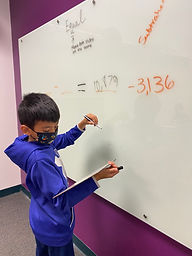## Ms. Briana

### Target 1​

###### Lesson Type:

New

Number Operation

:

Computation

Recognize and construct equivalent addition and subtraction equations (showing an understanding of the equal sign).

###### 1:

Define an equal sign as a symbol which shows that the quantity on both sides are the same.

###### 2:

Understand that an equal sign can link an equation to its solution or that it can link two equations together.

2nd

###### Vocabulary:

Equal, Equivalent, Construct and Quantity

Activities:

Students solved and matched premade equations on the large white board.

Students used playing cards to create the first part of differnet equations. Students then had to fill in the rest of the equation making sure that the answer to both equations was the same (started as group work and then moved into individual once the students were comfortable).### Home Exploration

###### Guiding Questions:## Absent Students:

### Target 2

:

###### 1:

Understand that multiplying numbers makes numbers larger.

###### 2:

Understand that multiplication represents the combination of equal groups of objects.

###### 3:

Understand that the same quantity can be group in multiple ways, creating different multiplication equations.

###### 4:

Understand that multiplication equations can be written both vertically and horizontally and multiplication is often identified by an “x” in an equation.

2nd

###### Vocabulary:

Vertically, Horizontal, Combination, Multiplication, Arrays and Skip Counting

Activities:

Students used the small number spinners and 6 sided dice to create multiplication equations.

Students used white boards to practice repeated addition method of multipliction solving.

Students worked together on the large whiteboard to solve double digit myltiplication problems. (worked together to master steps in standard form)### Home Exploration

###### Guiding Questions:### Target 3

:

###### Vocabulary:

Activities:### Home Exploration